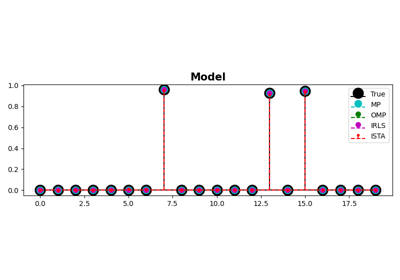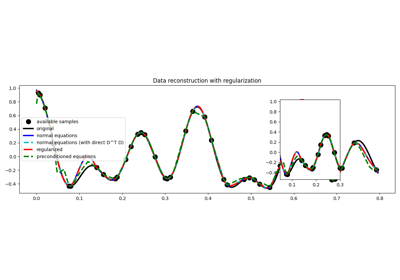# pylops.optimization.sparsity.ISTA¶

pylops.optimization.sparsity.ISTA(Op, data, niter, eps=0.1, alpha=None, eigsiter=None, eigstol=0, tol=1e-10, monitorres=False, returninfo=False, show=False, threshkind='soft', perc=None, callback=None, decay=None, SOp=None)[source]

Iterative Shrinkage-Thresholding Algorithm (ISTA).

Solve an optimization problem with $$L_p, \; p=0, 1/2, 1$$ regularization, given the operator Op and data y. The operator can be real or complex, and should ideally be either square $$N=M$$ or underdetermined $$N<M$$.

Parameters: Op : pylops.LinearOperator Operator to invert data : numpy.ndarray Data niter : int Number of iterations eps : float, optional Sparsity damping alpha : float, optional Step size ($$\alpha \le 1/\lambda_{max}(\mathbf{Op}^H\mathbf{Op})$$ guarantees convergence. If None, the maximum eigenvalue is estimated and the optimal step size is chosen. If provided, the condition will not be checked internally). eigsiter : float, optional Number of iterations for eigenvalue estimation if alpha=None eigstol : float, optional Tolerance for eigenvalue estimation if alpha=None tol : float, optional Tolerance. Stop iterations if difference between inverted model at subsequent iterations is smaller than tol monitorres : bool, optional Monitor that residual is decreasing returninfo : bool, optional Return info of CG solver show : bool, optional Display iterations log threshkind : str, optional Kind of thresholding (‘hard’, ‘soft’, ‘half’, ‘hard-percentile’, ‘soft-percentile’, or ‘half-percentile’ - ‘soft’ used as default) perc : float, optional Percentile, as percentage of values to be kept by thresholding (to be provided when thresholding is soft-percentile or half-percentile) callback : callable, optional Function with signature (callback(x)) to call after each iteration where x is the current model vector decay : numpy.ndarray, optional Decay factor to be applied to thresholding during iterations SOp : pylops.LinearOperator, optional Regularization operator (use when solving the analysis problem) xinv : numpy.ndarray Inverted model niter : int Number of effective iterations cost : numpy.ndarray, optional History of cost function NotImplementedError If threshkind is different from hard, soft, half, soft-percentile, or half-percentile ValueError If perc=None when threshkind is soft-percentile or half-percentile ValueError If monitorres=True and residual increases

OMP
Orthogonal Matching Pursuit (OMP).
FISTA
Fast Iterative Shrinkage-Thresholding Algorithm (FISTA).
SPGL1
Spectral Projected-Gradient for L1 norm (SPGL1).
SplitBregman
Split Bregman for mixed L2-L1 norms.

Notes

Solves the following synthesis problem for the operator $$\mathbf{Op}$$ and the data $$\mathbf{d}$$:

$J = ||\mathbf{d} - \mathbf{Op} \mathbf{x}||_2^2 + \epsilon ||\mathbf{x}||_p$

or the analysis problem:

$J = ||\mathbf{d} - \mathbf{Op} \mathbf{x}||_2^2 + \epsilon ||\mathbf{SOp}^H\mathbf{x}||_p$

if SOp is provided. Note that in the first case, SOp should be assimilated in the modelling operator (i.e., Op=GOp * SOp).

The Iterative Shrinkage-Thresholding Algorithms (ISTA)  is used, where $$p=0, 1, 1/2$$. This is a very simple iterative algorithm which applies the following step:

$\mathbf{x}^{(i+1)} = T_{(\epsilon \alpha /2, p)} (\mathbf{x}^{(i)} + \alpha \mathbf{Op}^H (\mathbf{d} - \mathbf{Op} \mathbf{x}^{(i)}))$

or

$\mathbf{x}^{(i+1)} = \mathbf{SOp}(T_{(\epsilon \alpha /2, p)} (\mathbf{SOp}^H(\mathbf{x}^{(i)} + \alpha \mathbf{Op}^H (\mathbf{d} - \mathbf{Op} \mathbf{x}^{(i)}))))$

where $$\epsilon \alpha /2$$ is the threshold and $$T_{(\tau, p)}$$ is the thresholding rule. The most common variant of ISTA uses the so-called soft-thresholding rule $$T(\tau, p=1)$$. Alternatively an hard-thresholding rule is used in the case of p=0 or a half-thresholding rule is used in the case of p=1/2. Finally, percentile bases thresholds are also implemented: the damping factor is not used anymore an the threshold changes at every iteration based on the computed percentile.

  Daubechies, I., Defrise, M., and De Mol, C., “An iterative thresholding algorithm for linear inverse problems with a sparsity constraint”, Communications on pure and applied mathematics, vol. 57, pp. 1413-1457. 2004.

## Examples using pylops.optimization.sparsity.ISTA¶MP, OMP, ISTA and FISTA03. Solvers